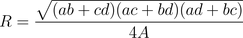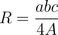## Saturday, 17 October 2009

I wrote recently about a trigonometric version of Heron's Formula, and most folks who have played with geometry beyond the basics of high school are probably aware of an extension called Brahmagupta's Formula,; where s is half the perimeter, s=which gives the area of a cyclic quadrilateral (one whose four vertices lie on a common circle.

I was just reading a journal article from the American Mathematical Monthly from 1922 that Brahmagupta was not aware that the formula was only true for cyclic figures. It was not until Bhaskara in the 12th century that this concept was proven.

In the same article it points out that if you have four distinct lengths for the four sides, they can be rearranged to make three different inscribable quadrilaterals, but all three will have the same area.In addition, although there are six different diagonals, but they only have three different lengths. In the image, the diagonals that have a common color are the same length. The lengths of the diagonals can be found by another interesting relationship. There are three ways to form a sum of the products of two of the legs, ab+cd, ac+bd, and ad+bc. These three values can be combined to make the lengths of the diagonals. In the first of the figures above (order abcd) a and c are the opposite sides, so the two diagonals will be given by :Note that the diagonal that goes from the intersection of sides a and b to the vertex where sides c and d meet, is given when ab+cd is in the numerator, and similarly for the other diagonal.

But what about the circle itself, how might we determine the radius of a circle that contains an inscribable quadrilateral with area A. Use these same three ratios all multiplied together, :If you treat a triangle as a degenerate cyclic quadrilateral (one side equals zero) then the last formula reduces to a somewhat well known formula for triangles, the circumcircle of a triangle is given by